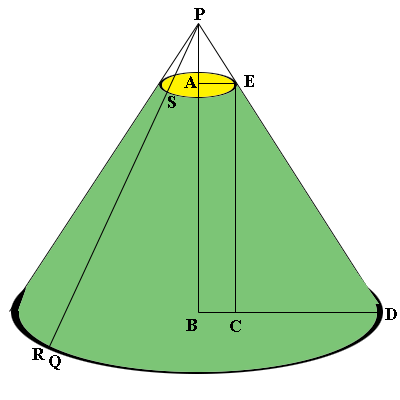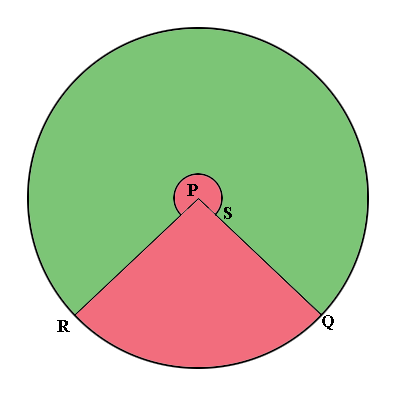SEARCH HOMEMath Central Quandaries & QueriesQuestion from Tom: I have researched several sites, including this one and am fairly confident I can do the calculations required to produce the two radii and the slant height for a truncated cone. I a somewhat confused by the central angle. Some sites indicate that it should never exceed 180 degrees while others do not. Different examples on your site seem to use this in two different ways in constructing a truncated cone. Sometimes the angle seems to indicate the section to be removed, while in other examples the angle seems to indicate the section to be saved. Since the two angles will always total 360 I am confused about how to use the info to calculate the minimum rectangle required to contain the pattern. I'm guessing that in some cases I may need to use the chord or sagitta to determine the desired height and width of the material. Any help is greatly appreciated. Thank you. TomHi Tom,

There is no reason that the central angle needs to measure less than 180 degrees.

I think that one of the examples on Math Central that you might have found confusing is my response to Fleur who was constructing a lampshade. I have copied the images from that page so that I can easily refer to them.The green lampshade is on the left and the template to form it is on the right. I used the green colour to indicate which part of the image on the right is to be used to form the lampshade. When I made the calculations I was focused on the pink piece to be removed so the central angle I calculated was the central angle of the pink section which turned out to measure 142 degrees. I could just as easily have calculated the central angle of the green segment on the right which, as you observed, would be 360 - 142 = 218 degrees. Let me do that calculation.

From my response to Fleur we know that $|PR| = |PD| = 33$ cm and $|BD| = 20$ cm. Thus the circumference of the base of the lampshade measures $2 \pi \times 20$ cm. On the template on the right this is the length of the arc measured counterclockwise from Q to R. Since $|PR| = 33$ cm the circumference of the outside circle on the right measures $2 \pi \times 3$ cm. Hence the central angle of the green sector on the right measures

$\frac{2 \pi \times 20}{2 \pi \times 33} \times 360 = 218 \mbox{ degrees.}$

I hope this helps,
PennyMath Central is supported by the University of Regina and The Pacific Institute for the Mathematical Sciences.